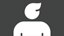cancel
Showing results for
Search instead for
Did you mean:Anonymous
Not applicable

# The loan manager interest calculation for 365/360 compute period is not correct?

I tested the loan manager with a loan: The borrowed amount is \$10000.00, term is 6 months, origination date is Dec/1/2018, due date of next payment is Jan/1/2019, payment amount is \$2000.00, payment period is monthly, interest rate is 36%, compounding period is Exact Days, computing period is 365/360. The interest amount that loan manager shows for the first payment is \$304.17. I don't think this is correct. My calculation is like this: the daily interest rate = 36%/360 = 0.001, so the interest for the first payment should be 0.001 * 10000.00 * 31 (days) = \$310.00. Am I correct?

Thanks.

2 CommentsCommunity Champion

## The loan manager interest calculation for 365/360 compute period is not correct?

Using http://www.amortization-schedule.info/calculator I get a monthly payment of \$1845.98 and first month interest is \$300 (36% of \$10,000 divided by 12 is exactly \$300) A payment of \$2000/month will retire the loan with a final 6th payment much less than the \$1846

Loan Manager rarely will match exactly what an actual bank calculates that you owe so in the end always refer to statement instead.Anonymous
Not applicable

## The loan manager interest calculation for 365/360 compute period is not correct?

http://www.amortization-schedule.info/calculator calculates interest for Monthly compounding period. What I want is interest calculation for Exact Days compounding period and 365/360 compute period. Can anyone show me this interest calculation?

Thanks.

## Need to get in touch?

Sitemap
Sign in for the best experience
Ask questions, get answers, and join our large community of QuickBooks users.
Sign In / Sign Up